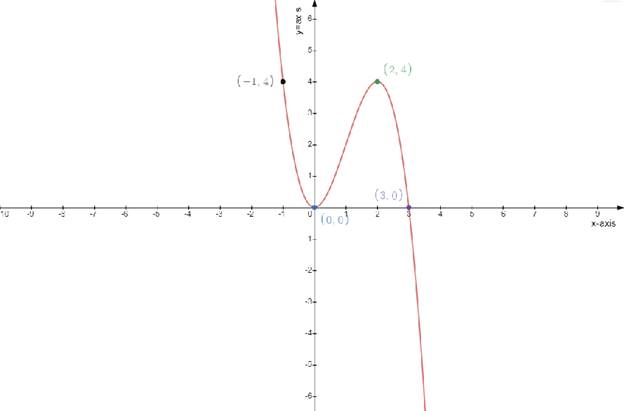# The equation of the tangent line to the curve at the given point### Single Variable Calculus: Concepts...

4th Edition
James Stewart
Publisher: Cengage Learning
ISBN: 9781337687805### Single Variable Calculus: Concepts...

4th Edition
James Stewart
Publisher: Cengage Learning
ISBN: 9781337687805

#### Solutions

Chapter 3.1, Problem 31E
To determine

## To find:The equation of the tangent line to the curve at the given point

Expert Solution

The equation of the tangent line is y=3x(2x)(x1)+2

### Explanation of Solution

Given:

The function is

f(x)=3x2x3

Concept used:

An equation of a line l through a point P1(x1,y1) with slope m . If P(x,y) is any point with xx1

Then P is on l if and only if the slope of the line through P1 and P

The equation is yy1=m(xx1)

Calculation:

The function

f(x)=3x2x3...................(1)

The derivative of a function

y=f(x)y=f(x)=dydx

Differentiating the equation (1) with respect to x

dydx=ddx(3x2x3)dydx=6x3x2dydx=3x(2x)

The point is (1,2)

The equation is

yy1=m(xx1)y2=3x(2x)(x1)y=3x(2x)(x1)+2

Draw the table

y=f(x)=3x2x3

Test one point in each of the region formed by the graph

If the point satisfies the function then shade the entire region to denote that every point in the region satisfies the function

 x−axis 0 1 2 3 y−axis 0 4 4 0### Have a homework question?

Subscribe to bartleby learn! Ask subject matter experts 30 homework questions each month. Plus, you’ll have access to millions of step-by-step textbook answers!